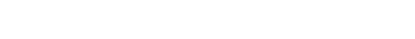Different ionic compounds can release the same ions into solution. This ultimately means that different ionic compounds can affect the equilibrium of other ionic compounds.

Common ion effect: The solubility of a salt can be reduced by the presence of another salt that has a common ion.

Example: if NaCl is dissolved and HCl(aq) is added to the solution. This introduces chlorine ions and forces the equilibrium to the left due to Le Châtelier’s Principle, producing more NaCl(s).Practice: If KBr is added to a solution containing PbBr2(aq), will more PbBr2 solidify? Yes

If KBr is added to a solution containing NaCl, will more NaCl precipitate? No, no common ion

Example: How many moles of PbCl2(s) will dissolve in 1L of 0.2M NaCl at SATP?

Common ion = Cl-1

[Cl-1]=[NaCl]=0.2MSince there are no lead ions to start, Q=0 and the rxn shifts to the right

I     –           0          0.2

C    –         +x         +2x

E    –           x       0.2+2xSubscribe
Notify of